# Gate Mock Test- 15: CS/IT

## 65 Questions MCQ Test GATE Computer Science Engineering(CSE) 2023 Mock Test Series | Gate Mock Test- 15: CS/IT

Description
Attempt Gate Mock Test- 15: CS/IT | 65 questions in 180 minutes | Mock test for GATE preparation | Free important questions MCQ to study GATE Computer Science Engineering(CSE) 2023 Mock Test Series for GATE Exam | Download free PDF with solutions
QUESTION: 1

### A 300-meter-long train passes a 450-meter-long platform in 5 sec. If a man is walking at a speed of 4 m/sec along the track and the train is 100 m away from him, how much time will it take to reach the man?

Solution:

The train can cover (300 + 450) m distance in 5 sec.

The speed of the train = 150 m/sec

Relative speed of the man and the train is 154 m/sec or 146 m/sec

To cover the distance of 100 m, in either of the case, it will take less than 1 second.

Hence, (C) is the correct option.

QUESTION: 2

### A boat takes half the time moving a certain distance downstream than upstream. The ratio of the speed of the boat to that of the current is

Solution: Let,

Boats speed = x kmph

Current speed = y kmph

Time taken in upstream = t hours

Therefore, (x - y) × 2t = (x + y) × t

⇒ 2x – 2y = x + y

⇒ x = 3y ⇒ x : y = 3 : 1

QUESTION: 3

### Which of the following word is opposite to the word “connivance”?

Solution:

Connivance- willingness to allow or be secretly involved in an immoral or illegal act

Conspiracy- a secret plan by a group to do something unlawful or harmful.

Sufferance - capacity to endure pain, hardship, etc.; endurance

Complot - a plot involving several participants; conspiracy

Intolerance - unwillingness or refusal to tolerate or respect persons of a different social group, especially members of a minority group.

QUESTION: 4

Direction: A number is wrong in the following number series. Select the wrong number.

64, 130, 264, 536, 1076

Solution: The series is

64 × 2 + 2= 130

130 × 2 + 4= 264

264 × 2 + 6= 534

534 × 2 + 8 = 1076

QUESTION: 5

Direction: Each statement has a blank followed by four options. Select the most appropriate word for the blank.

Guru was always able to maintain a _______ face when he said something silly, and that contrast made everyone laugh.

Solution: The statement means to state that Guru had an ability to maintain a serious face while saying something silly. The biggest hint in the question is the word ‘contrast’. Hence, c is the correct answer.
QUESTION: 6

A supermarket launched a scheme that if a customer purchases two CDs, one extra CD will be free and if he purchases 3 Mobile he will get one extra Mobile free. If the cost price of 3 CD and 4 Mobile be Rs.6700 and Rs.232500 respectively. If a customer purchase 2 CD and 3 Mobile as per scheme he availed 1 product free of each category, then at what price these product should be sold so that the agency can get overall profit of 17.5%

Solution:

CP of 2 CD and 3 Mobile = 6700 + 232500 = 300000

Now, since we required 17.5% profit,

So, SP= 300000*117.5/100 = Rs. 352500.

QUESTION: 7

In the following question, two/three statements are given followed by four conclusions. You have to consider the statements to be true even if they seem to be at variance from commonly known facts. You have to decide which of the given conclusions, if any, follow from the given statements.

Statements:

I. Some cats are owls.

II. Some owls are elephant.

Conclusion:

I. Some cats are elephant.

II. All owls are elephant.

Solution:

Here two possibilities emerge based on statement I and II.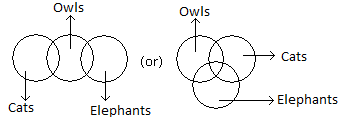Conclusion I:- It cannot be said with certainty that some cats are elephants.

Conclusion II: - It cannot be said with certainty that all owls are elephants.

Hence, neither conclusion I nor II follows.

QUESTION: 8

In the following question, a set of labelled sentences is given. Out of the four alternatives, select the most logical order of the sentences to form a coherent paragraph.

P) The over 11-km Mundka-Bahadurgarh section of Delhi Metro’s Green Line, connecting the capital of Haryana, was inaugurated by the Prime Minister.

Q) The rapidly growing industry in the area was waiting for Metro connectivity, said Mr.PM, adding that it would create new employment opportunities and benefit students.

R) Built at a cost of Rs 2,028 crore, the Mundka-Bahadurgarh elevated section, with seven stations, is the third Metro extension between Delhi and Haryana.

S)Mr. Prime Minister said Bahadurgarh was known as the Gateway of Haryana and would now be the Gateway of Development with the advent of the Metro.

Solution:

The given passage talks about the plan of a metro, hence P should be the first sentence as it states about the initiation of the plan. R gives another basic information about the plan, i.e. its cost etc. Thus, R should be the second sentence. Sentence S must follow next because Q contains the abbreviation which is mentioned in sentence R. Hence, option A is correct.

QUESTION: 9

Study the information given below and answer the questions based on it.

Eight friends, A, B, C, D, E, F, G and H are sitting around a circle (not necessarily in the same order) facing the centre. B sits third to the left of F. E is an immediate neighbour of both B and H. Only one person sits between A and H. C and G are immediate neighbours of each other. Neither C nor G is an immediate neighbour of B. Only one person sits between C and D.

Who amongst the following is an immediate neighbour of both A and H?

Solution:

From the given seating arrangements, we can conclude as follows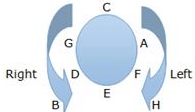Hence F is an immediate neighbour of both A and H.

QUESTION: 10

Direction: Study the line graph carefully and answer the given questions.

The graph shows that the total number of students attended the exams and the total number of students passed in the exam in different years of a school.

Note:

1. The total number of students enrolled in each year= The total number of students who appeared in the exam + The total number of students who didn’t appear in the exam

2. The total number of students who appeared in the exam= The total number of students passed in the exam + The total number of students who failed in the exam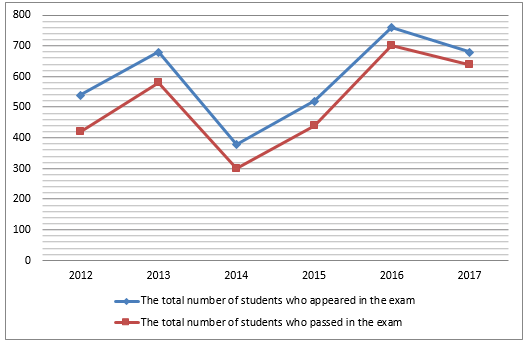What is the average number of students failed over the years?

Solution: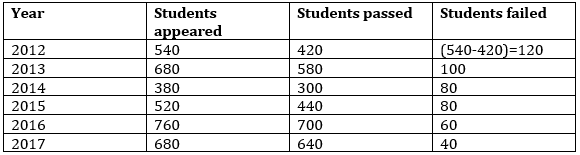Total number of students failed over the years = 120 + 100 + 80 + 80 + 60 + 40 = 480

So, required average=480/6=80

QUESTION: 11

S={1,2,...,n} of n unit-time tasks with deadlines {d1, d2, ..., dn} and penalty(non –ve) {w1, w2 ,..., wn} for missing the deadlines. Objective is to find a schedule for S that minimizes the total penalty. Execution of each task requires one unit of time. Given below is a set of tasks:

Solution: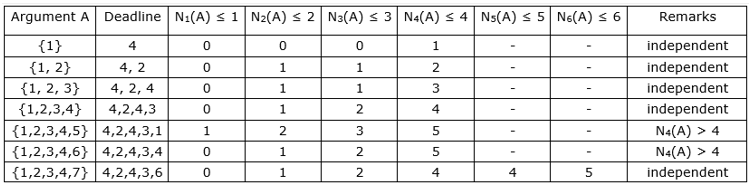The set S={1,2,3,4,5,6,7} of tasks is sorted on the penalty.

For t=1,2,..,n; let Nt(A) denote no of tasks in A whose di ≤ t i.e. deadline is t or earlier. Clearly, if Nt(A) > t for some t, then there is no way to make a schedule with no late tasks for set A, because there are more than t tasks to finish before time t.

● Next, we sort A on deadlines so that schedule is early tasks <2,4,1,3,7> followed bylate tasks <5,6>.

11-35

QUESTION: 12

Consider the following statements regarding Eigenvalues:

S1: Eigenvalues of A and A-1 are the same.

S2: Eigenvalues of A and AT are the same.

S3: If A is a singular matrix, then at least one of its Eigenvalue is 0.

Which option is correct?

Solution:

S1: The Eigenvalues of A 1 are the reciprocal of Eigenvalues of A, Hence False

S2: The Eigenvalues of AT are the same as of Eigenvalues of A, Hence True

S3: If A is singular matrix, then |A| = 0. As the determinant is given by the product of Eigenvalues, hence for the product to be 0, at least one of the Eigenvalue must be 0.

Hence S2 and S3 are correct.

QUESTION: 13

Consider a new data structure which is named as A- tree. Consider the properties of new discovered A-tree:

• The nodes are inserted in such a way that all the nodes in the left subtree of a particular node are smaller than that node. Also, all the nodes in the right subtree of a particular node are larger than that node

• The height of the tree is balanced in such a way that the height of the left subtree and height of the right subtree differ by almost one.

Let the minimum number of nodes required to generate an A- tree of height 6 = 'a' and the maximum number of nodes which can be there in A- tree of height 6 = 'b' , find |a-b| = ________.

Solution: Let h(n) be the minimum no. of nodes required by AVL tree of height n.

for height (O) h(n) = 1 (root node)

height (l) h(n) = 2 height (2) h(2) 4

Also, h(n) = h(n-1) + h(N-2) + 1

for height 6.

h(3) = h(1) + h(2) + 1 = 7

h(4) = h(3) + h(2) + 1 = 12

h(5) = h(4) + h(3) + 1 = 20

h(6) = h(5) + h(4) + 1 = 20 + 12 + 1 = 33

value of a = 33

Also, no. of Nodes will be maximum when tree is a complete binary tree

No. of Nodes in a complete tree with height (n) = 2n+1 - 1

= 27 – 1 = 127

value of b = 127

|a-b| = 127 – 33 = 94

QUESTION: 14

Which of the following statements about parser is/are CORRECT?

I. Canonical LR is more powerful than SLR

II. SLR is more powerful than LALR

III. SLR is more powerful than Canonical LR

Solution: Bottom up parsers in decreasing order of their power: CLR≫ LALR≫ SLR≫ LR (0)

The given statements:

I. Canonical LR is more powerful than SLR is CORRECT.

II. SLR is more powerful than LALR is INCORRECT

III. SLR is more powerful than Canonical LR is INCORRECT.

QUESTION: 15

Which algorithm is mainly used in military applications to send and receive messages.

Solution: Military applications use flooding algorithms as during war routers may get damaged or get exploded, in flooding algorithm message is forwarded through all available paths so it gives guarantee that message will surely reach the destination.
QUESTION: 16

Given a disconnected graph on 10 vertices, what is the max number of edges it can have ________.

Solution: Maximum number of edges in a graph with 'n' vertices =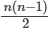Minimum number of edges to be removed to make the graph disconnected = (n-1)

Hence overall maximum number of edges in a disconnected graph =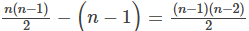Hence for 10 vertices, (9 x 8) / 2 = 36

QUESTION: 17

C What does the following fragment of c program print?

#include

int main()

{

static int GATE[]={100,200,300,400,500};

static int *ptr = {GATE+2,GATE,GATE+3,GATE+4,GATE+1}

int **p = ptr;

ptr++;

printf("%d%d", ptr - p, **ptr};

}

The output of the program is______

Solution: In order to simplify programs involving complex operations on pointers, we suggest you to draw proper diagrams in order to avoid silly mistakes. Let’s assume that integer is of 4 Bytes and Pointer size is also 4 Bytes.

Let’s assume array a Base address is 1000. Array name actually holds the array base address.

Array A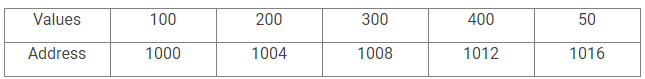Let’s assume array p Base address is 2000.

Array P.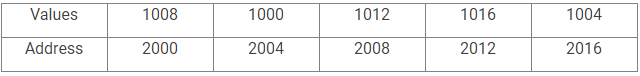Double Pointer ptr Base Address is 3000.

Ptr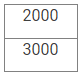Now ptr is actually pointing to the first element of array p. ptr++ will make it point to the next element of array p. Its value will then change to 2004.

One of the Rule of Pointer Arithmetic is that When you subtract two pointers, as long as they point into the same array, the result is the number of elements separating them.

ptr is pointing to the second element and p is pointing to the first element so ptr-p will be equal to 1(Excluding the element to which ptr is pointing).

Now ptr = 2004 →*(2004) = 1000 →*(1000) →100.

Therefore, the final answer is 1100.

QUESTION: 18

Assume host A having an IP address of 125.32.16.5 with a subnet mask of 255.255.255.128. Also, assume another host B, having an IP address of 125.32.16.120 with a subnet mask of 255.255.255.192. Which of the following is correct?

Solution: IP address of 'A' = 125.32.16.5

Subnet mask of 'A' = 255.255.255.128

Subnet ID of A according to 'A' = 125.32.16.0

IP address of B' = 125.32.16.120

Subnet ID of B according to A' = 125.32.16.0

Hence 'A' assumes 'B' to be on the same network

IP address of B' = 125.32.16,120

Subnet mask of 'B' = 255.255.255.192

Subnet ID of B according to 'B' = 125.32.16.64

Subnet ID of A according to 'B' = 125.32.16.0

Hence 'B' assumes 'A' to be on a different network.

QUESTION: 19

Consider the following statements:

S1: The number of relations which are both reflexive and asymmetric is 0

S2: The number of relations which are both symmetric and asymmetric is 0

Which option is correct :

Solution:

S1: No relation is possible which is both reflexive and asymmetric because to be reflexive, all self-loops must be there but asymmetric doesn’t allow self-loops. Hence 0 relations.

S2: Only a single relation, i.e. empty relation is both symmetric and asymmetric.

So only S1 is correct.

QUESTION: 20

A system Jarvis requested for a specific site "abc.co", in return to this request it got the error as "LOOKUP FAILED". Now consider the following services:

1) SMTP

2) TCP

3) UDP

4) DNS

Which of the above service(s) are failed when we get the error as "LOOKUP FAILED"

Solution: Lookup failed means DNS has failed. The conversion from website name to its IP address can't be done.
QUESTION: 21

Consider a binary tree in which every node has 3 attributes (Left child pointer, DATA, right child pointer). The tree has 3 levels. Now consider the following three cases:

Case 1: The tree is completely filled

Case 2: The last level of the tree is half-filled

Case 3: The last level of the tree is quarterly filled

If the total number of pointers in case 1 = a

similarly, the total number of pointers in case 2 = b

and, the total number of pointers in case 3 = c

Find the value of (b+c-a).

Solution:

Case 1: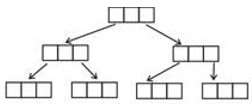Total pointers, a = 14

Case 2: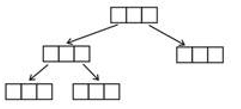Total pointers, b = 10

Case 3: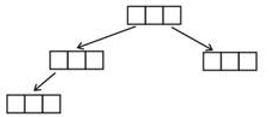Total pointers, c = 8

(b+c-a) = 10 + 8 – 14 = 4

QUESTION: 22

Consider a Demand Paging Environment Where the system requires 4 bytes to store integers and page size is of 256 bytes.

Assume the given code.

Int a [][] = new int a;

Int i=0, j=0;

While (i++ < 200)

{

while (j++< 250)

a [i][j]= 0;

}

What is the number of pages referenced from the system while executing the above code?

Solution: Here, page size= 256 B

Integer Size= 4 B

No of integers on a single page= 256/4 = 64

Total no of integers to be stored in array= 200* 25 = 50000

Therefore, the total no of pages= no of integers/ integers per page

= 781.28

= 782 pages.

QUESTION: 23

Consider the following statements:

S1 : When in any application the number of insertion are very large but the number of deletion are very less. Then unordered array data structure give better performance than ordered array data structure.

S2 : When in any application number of insertion and number of deletion are same then binary search tree data structure give better performance than max heap data structure.

Which of the following is correct?

Solution: S1 : Since number of insertions are high, hence in unordered array every insertion can be done at end which will take O(1) time, but in an unordered array, all the insertions will be done somewhere in there right positions which will take O(log n) time. Hence an unordered array will give better performance than ordered array data structures.

S2: All the operations in a binary search tree takes O(h) time where the height can go till O(n), which is not the case in max heap. Hence the statement is false:

QUESTION: 24

A secondary index on a non candidate key

Solution: Secondary index may be from a field which is a candidate key and has a unique value in every record, or a non-key with duplicate values. Here the secondary key on a non candidate key will have duplicates.

Since the search key of the secondary index is not a candidate key and the file is unordered, only one record pointer per search key is not enough. There must be record pointer for each record. Hence dense index.

QUESTION: 25

Consider the following elements:

5 , 12 , 3 , 15 , 4 , 6 , 10

These elements are inserted one-by-one into two separate heaps, one min-heap and another max heap. Then these two heaps are stored in two different arrays starting from index How many elements have the same index value in both the arrays _______.

Solution:

Min-Heap after all insertions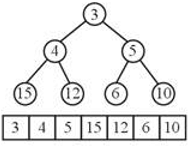Max-Heap after all insertions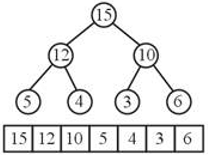Clearly, no element has the same index.

QUESTION: 26

The trace and determinant of a 2 × 2 matrix are known to be –2 and –35 respectively. Its eigenvalues are :

Solution: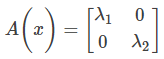∴ Trace = λ1 + λ2 = -2

Determinant = λ1 λ2 = -35

Solving , we get λ1 = -7, and λ2 = 5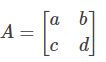∴ a + d = -2

and ad – bc = -35

Alternately

Now,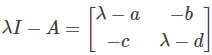= (λ – a) (λ – d) – bc = 0

λ2 – (a + d) λ – bc + ad = 0

λ2 + 2λ – 35 = 0

⇒ (λ + 7) (λ – 5) = 0

λ = -7 or λ = 5

QUESTION: 27

Assume that the time required for the eight functional units, which operate in each of the eight cycles, are as follows

5ns, 8ns, 6ns, 10ns, 15ns, 12ns, 6ns, 8ns

Assume that pipelining adds 1ns of overhead. Find the speedup versus the single cycle datapath.

Solution: Since the unpipelined machine executes all instructions in a single clock cycle, its average time per instruction is simply clock time. The clock is equal to the sum of the times for each step in the execution

Average instructions execution time = 5 + 8 + 6 + 10 + 15 + 12 + 6 + 8 = 70

The clock cycle time on the pipelined machine must be the largest time for any stage in the pipeline (15 ns) + the overhead of 1 ns for a total 16ns

Speed for the pipelining = Average instruction time unpipelined / An instruction time pipelined

= 70ns / 16ns

= 4.375 times.

QUESTION: 28

If the connection of the input 3×8 decoder is as shown in the figure, then the output F(A,B,C) can be expressed as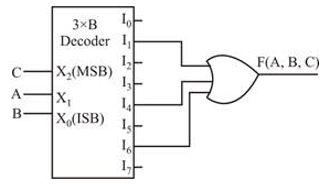Solution:

From the decoder circuit it is clear that F(A,B,C)= I1+I4+I6

Where I1 = C’A’B

I4 = CA’B’

I6 = CAB’

But till now the function is expressed as F(C,A,B) as C is the MSB in the given decoder. So rearranging the terms, we get -

I1 = A’BC’ = 2

I4 = A’B’C = 1

I6 = AB’C = 5

Therefore, F(A,B,C) = Σm(1,2,5)

QUESTION: 29

Consider the disk with following characteristics. Computer Network

• Transfer speed of 107 bytes/sec

• 10,000 RPM

• Average seek time of 8ms

• Each disk operation has 2 ms overhead, Assume system bus has a maximum bandwidth of 133 Megabytes per second and network connection has bandwidth of 107 bytes/sec. HTML files have an average of 8000 bytes. Each HTML file occupied one sector.

Find the throughput to transfer HTML files? (Round to nearest integer). Assume throughput is the number of HTML files read per second?

ptions~

(a) 72

(b) 73

(c) 75

(d) 76

Solution: Time taken to transfer one HTML file

=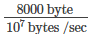= 0.8 ms

Avg. rotational latency = ½ x 6ms = 3 ms

[10,000 RPM ⇒1 rotation = 6 ms]

∴ Avg time to read an HTML file

= 0.8 + 3 ms + 8 ms + 2 ms = 13.8 ms

Time taken to read one HTML file = 13.8ms

1 sec ⁡⇒ 1 sec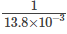= 72 files

∴ 72 HTML files can read per second.

QUESTION: 30

Consider a B+ tree in which the maximum number of keys in a node is 8. What is the minimum number of keys present in a non-leaf node?

Solution: maximum pointer = keys + 1 (order) = 9

minimum pointer = 9 / 2= 5

minimum keys = 4

QUESTION: 31

Which of the following is not a token in C language.

Solution:

C tokens are:

I. Keywords

II. Identifiers.

III. Constants.

IV. Symbols (;, (, ), }, {, etc)

V. Operators.

White spaces are used to separate the tokens but not counted as a token. White spaces are ignored while identifying the tokens.

QUESTION: 32

Consider a 4-bitJohnson counter with an initial value 1111. Then the counting sequence of this countries:

Solution: The Johnson counter complements the last digit and feeds it to the first digit. 1111 → 15 (1 complemented and then added as first digit in the next iteration) 0111 → 7 (1 complemented and then added as first digit in the next iteration) 0011 → 3

0001 → 1

0000 → 0 and so on…

QUESTION: 33

The shift register shown in the figure is initially loaded with the bit pattern 1010. Subsequently the shift register is clocked and with each clock pulse the pattern gets shifted by one bit position to the right. With each shift, the bit at the serial input is pushed to the left most position (msb). After how many clock pulse will the content of the shift register become 1010 again?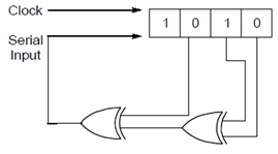Solution: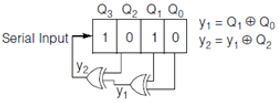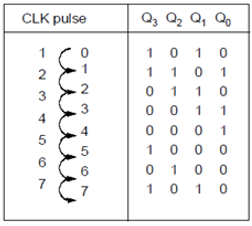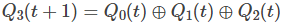QUESTION: 34

Let the two boolean functions as shown below -

F = AB'C + AB + A' + B' + C'

G = A'BC' + A'B + A'B' + B'

Which of the following is true regarding the above functions?

Solution:

F = AB'C + AB + A' + B' + C'

= A[B'C+B] + A' + B' + C'

= A[B+C] +A' + B' + C' {DISTRIBUTIVE PROPERTY}

= A' + B + C + B' + C'

= T

Now, for function G-

G = A'BC' + A'B + A'B' + B'

= A'[BC' + B + B'] + B'

= A'[BC' + 1] + B'

= A' + B'

= (A.B)'

Which is a NAND gate so the function {G} is functionally complete.

QUESTION: 35

Throughput of network is dependent on –

Solution: Throughput of a network is actually how much effective bandwidth is being used in a network.

Effective bandwidth or Throughput =BW* efficiency

Efficiency is how much effective work is being done. This efficiency of the network is dependant of Data bit rate of the network, distance between two hosts, quality of host's processor, all these.

QUESTION: 36

Consider a system with a pipeline to provide speedup of 6.2 at 100MHz and has 70% efficiency. Find the number of stages in the pipeline.

Solution:

[(Speedup)/number of stages] × 100 =efficiency

Number of stages = 6.2 ×100/70 = 8.8 →9

QUESTION: 37

Construct a B+-tree for the following set of key values:

(2, 3, 5, 7, 11, 17, 19, 23, 29, 31)

Assume that the tree is initially empty and values are added in ascending order. Construct B+-trees for the cases where the number of pointers is 4 and tell which element is the root node of the tree.

Solution: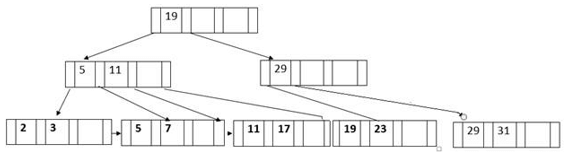QUESTION: 38

Which of the statements are true?

II. Protected members are accessible to the members of derived classes.

III. A derived class inherits constructors and destructors.

IV. A friend function can be called like a normal function.

V. Nested class is a derived class.

Solution:

When you using Overloading, you are using static (compile-time) polymorphism because the compiler is aware of exactly which method you are calling. Private members are only accessible within the class defining them. Protected members are accessible in the class that defines them and in classes that inherit from that class.

QUESTION: 39

Consider a disk with block size B = 512 bytes. A block pointer is P = 6 bytes long, and a record pointer is PR =7 bytes long. A file has r = 16384 STUDENT records of fixed-length. Each record has the following fields:

NAME (30 bytes), ROLL(9bytes), DEPARTMENT(9 bytes), ADDRESS(40 bytes),

PHONE (10 bytes), DOB(8 bytes), CLASS(4 bytes),

Two additional bytes are used as markers. Multilevel indexing is used.

If the file is ordered using ROLL value, the number of block accesses needed to search for and retrieve a record from the file is:

Solution:

Record length(R) = 30 + 9 + 9 + 40 + 10 + 8 + 4 + 2 = 112.

Blocking factor(bfr) = floor(B/R) = floor(512/112) = 4.

No. of blocks needed for data = ceil(16384/4) = 4096.

Index record size = P+ = 6+9 = 15

(Since primary indexing, file is ordered using ROLL key, so sparse index, so block pointer is used; not record pointer).

index blocking factor(bfri) = floor(512/15) = 34.

No. of first level index entries = no.of first-level blocks = ceil(4096/34) = 121.

No. of second level index entries = no.of second-level blocks = ceil(121/34) = 3.

No. of third level index entries = no.of third-level blocks = ceil(3/34) = 1.

So no. of levels obtained above for multilevel indexing = 3

So no of block access = no of index block accessed for each level + data block access by block pointer = 3 + 1 = 4.

QUESTION: 40

Given below is a system of linear equations.

5a + b + 3c = 5

9a + c = -8

a + 2b + 5c=8

This system of equations has,

Solution:

Comparing the system of equation with the matrix equation,

A.X = B

We have,

A =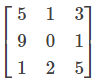, B =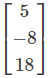Augmented matrix is given by,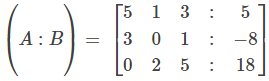Applying, R3→R3−(2R1−R2)

We get,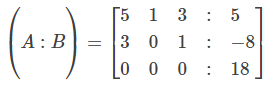Thus, Rank(A)=Rank(A:B)=2 < />

Hence, the system of equations is consistent and has infinitely many solutions.

QUESTION: 41

Given a relation is in 3NF, Which of the following can be inferred from this?

Solution:

Each non key attribute is determined by the super key,

A relation C-> D is in 3NF if,

1) C is a super key

or

2) D is a prime attribute

If D is a non prime attribute then C must be a super key.

QUESTION: 42

An electronic safe has a key which is made up of 5 digits (not necessarily distinct). It is also known that the first digit is three times the last digit. The third digit is 3 less than the second digit and the fourth digit is 4 more than the second digit. Also there are 3 pairs of digits which add up to give 11. The 5 digit number which can open the electronic lock of the safe is______

Solution:

A B C D E

3E B B-3 B+4 E

3 0 7 ……… (I)

4 1 8 …… (II)

5 2 9 ……. (III)

3 1…(IV)

6 2….(V)

9 3…(VI)

In order to satisfy the other condition that pairs of digits add up to give 11. Based on inputs, out of the possible sets listed above, we can the ONLY possible set fulfilling given conditions formed by combining III and V giving us the unique code as 6 5 2 9 2

6 + 5=11=2+9=9+2.

QUESTION: 43

Consider a client server system which uses satellites for their communication. Satellite is located at a height of 30,000 Km. Find the best case delay in response to a request.

Solution: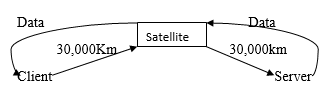= Client T.D. + P.D.(client to satellite) +P.D.(sat to server) +P.D.(server to sat) +P.D.(sat to client)

= 0 +(30000+30000+30000+30000)/3*108=120000/3*108(speed of light)

= 4 × 10-4 = .4 ms.

QUESTION: 44

Assume an instruction set that uses fixed 20 bit instruction length operands are 8 bits in length. There are P two operand instructions and Q zero operand instructions. Find the maximum number of one operand instruction that can be supported.

Solution:

Let x be the number of 1 operand

Total op-codes possible =16.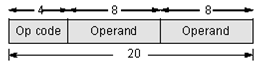Remaining op-codes for 1 operand instruction=(16-P)

Possible 1 operand instruction=(16-P)28

Remaining op codes for zero operand instruction = (16-P) x 28 - x

Possible zero operand instruction

= ((16-P)28 – x) 28 = Q

1 operand instruction is maximum when P=Q=0

((16 - 0)28 - x)28 = 0

1628 = x

x = 212 = 4096.

QUESTION: 45

For the given instructions, all types of data dependencies(RAW, WAW, WAR) are present.

STORE R1, 0[R2]

STORE R3, -8[R9]

Then which of the following is true?

Solution:

From the given instructions clearly,

- RAW exists if (R2+0) == (R8+24)

– WAR exists if (R8+24) == (R9 – 8)

– WAW exists if (R2+0) == (R9 – 8)

QUESTION: 46

Consider the following “Max-heapify” algorithm.Array has size at least n and 1in.After applying The Max-heapify noded at A[i], the result will be the subtree of A[1,….n] rooted at A[i] is a max-heapify.{ Assume that except root A[i], all its children satisfies heap property]

Max –heapify (int A[ ], int n,int i)

{ int p,m;

p = i;

while (2pn)

{

if(Y && Z)

m = 2p+1;

else m =2p;

if(A[p]

[swap (A[p],A[m]);

p=m;

}

else

return ;

}

}

Find missing statements at Y and Z respectively to apply the heapify for subtree rooted at A[i].

Solution: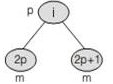If A[2p+1]>A[2p] then m=2p+1

else m=2p Y is :(2p+1)≤n

Z is :A[2p+1]>A[2p]

So, option B is correct.

QUESTION: 47

An AVL tree is constructed by inserting the following sequence of elements into empty AVL tree. After building an AVL tree, if an element ‘4’ is deleted from it, find how many levels are present in the AVL tree.

7, 4, 9, 5, 4.5, 6, 5.5, 5.2

Solution:

7, 4, 9, 5, 4.5, 6, 5.5, 5.2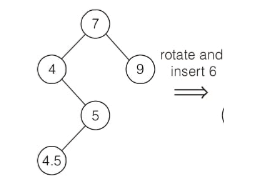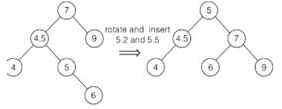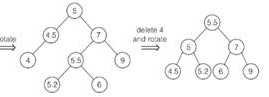Thus, 3 levels are present in the final AVL tree.

QUESTION: 48

The graph shown below has distinct edge weights and MST of weight 40 and MST contain edges

{(A, C), (B, C), (B, E), (E, F), (D, F)}. The minimum possible sum of weights of all 8 edges of this graph is _____.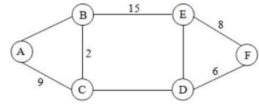Solution:

By using principle of Kruskals algorithm, to construct MST, we have to include edges in increasing order of their weights.

Step 1: DE > EF and DE > DF

∴ Minimum possible value of DE = 9 but graph has distinct edge weight so DE = 10.

Step 2: AB > AC and AB > BC

∴ Minimum possible value of AB = 10 [But we have DE = 10,

So, take AB = 11]

Step 3: DC > BC, DC > BE

Minimum possible value of DC = 16

∴ Sum of weights of all edges

= 9 + 2 + 15 + 8 + 6 + 16 + 10 = 77

QUESTION: 49

Let L={wε(0+1)*| w has even number of 1s}, i.e. L is the set of all bit strings with an even number of 1s. Which one of the regular expressions below represents L?

Solution:

Every string should contain an even number of 1 's min strings are ∈0,11,101, 1010,0101,0110,…

We can't get it by 0∗(10∗1)0∗ and 0∗1(10∗1)∗10∗

because these don't give ∈ and 0 strings.

QUESTION: 50

Consider the following schedule:

S: r2(z),r2(y),w2(y),r3(y),w3(z),r1(x),w1(x) ,w3(y),w3(z),r2(x),r1(y), w1(y),w2(x)

Which of the following is true about above schedule S.

Solution:

The precedence graph of the given schedule is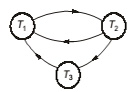There is a cycle in the precedence graph, the schedule is not serializable.

QUESTION: 51

Consider the following instruction sequence:

I1: R1 = 100

I2: R1 = R2 + R4

I3: R2 = r4 – 25

I4: R4 = R1 + R3

I5: R1 = R1 + 30

The number of RAW, WAR, WAW dependencies are

Solution:

No of dependencies can be represented as follows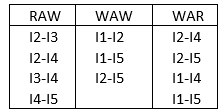So option C is correct.

QUESTION: 52

Consider the following 8-bit data computation:

(-121) + (-63)

What is the status of carry, parity, sign, and overflow flags in the computation respectively?

Solution:

2’s complement of (-121) = 10000111

2’s complement of (-63)= 11000001

1 01001000

Here, Carry=1, Sign=0, Parity=0, Overflow= 1

QUESTION: 53

Consider the following statements about graph

(1) The number of perfect matchings possible for K2n is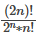(2) The chromatic number of the cyclic graph is 2, when the graph consists of an odd number of vertices.

(3) Consider a graph G, X is an independent set in G iff (V(G) – X) is a vertex cover of G.

(4) A bipartite graph with 2n vertices will have a maximum number of edges when both the partitions have equal number of vertices.

The number of incorrect statements is _________________?

Solution:

Statement 1 is true.

Statement 2 is false, because when the cyclic graph consists of an odd number of vertices we require at least 3 colors to cover them. You try to colour a cyclic graph with odd vertices and you will find it.

Statement 3 is true. Statement 4 is also true.

QUESTION: 54

Maximum port present in NIC card for –

Solution:

In mesh topology each host device is connected to all other host devices in that network. Hosts are connected through port of NIC card. So more hosts in a mesh topology network more port required in NIC card.

QUESTION: 55

You have a network 192. 168. 10. 0/24. How many subnets and hosts are available?

Solution:

192. 168. 10. 0/24 is a class C network using a default mask. This provides a simple single network with 254 hosts.

QUESTION: 56

How long does it take a packet of length 1,000 bytes to propagate over a link of distance 2,500 km, propagation speed 2.5 * 108 m/s, and transmission rate 2 Mbps? Does this delay depend on packet length? Does this delay depend on transmission rate?

Solution:

here, length of link= 2500 Km

Propagation Speed= 2.5 × 108 m/s

Propagation delay= 2500 × 103/ 2.5 * 108 = 10 msec

Also, Propagation delay is independent of packet length as well as transmission rate.

QUESTION: 57

Consider the following network establishment :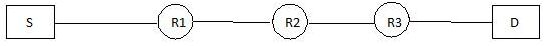How many times the network layer and data link layer is visited by the packet transmitted from source S to destination D, where R1, R2 and R3 are the destination routers?

Solution:

Since Router is a 3 layer Switch.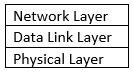When a packet visits the router it first go upto network layer and then retransmitted to the next router then, the data link layer is visited twice on a particular network and the network layer is visited once.

Hence, No of times network layer is visited= 3 + 2 (At sender and receivers End) = 5

No of times the data link layer is visited= 3 × 2 + 2 = 8.

QUESTION: 58

The least number of temporary variables required to create a three-address code in static single assignment form for the expression a*b+c-d^f*g is__________________.

Solution:

In compiler design, static single assignment form (often abbreviated as SSA form or simply SSA) is a property of an intermediate representation (IR), which requires that each variable is assigned exactly once, and every variable is defined before it is used. Existing variables in the original IR are split into versions, new variables.

We will need a temporary variable for storing the result of each binary operation as SSA (Static Single Assignment) implies the variable cannot be repeated on LHS of assignment.

t1 = d^f

t2 = a*b

t3 = t1*g

t4 = t2-c

t5 = t4-t3

Hence 5 temporary variables required.

QUESTION: 59

Consider the following grammar :

S→ aAb | Sc

A→ d | Sd | S

The above grammar is:

Solution:

⇒ as the given grammar is left recursive (S->Sc), so it is not LL(1) grammar.

⇒ as the given grammar has SR conflict,it is not LR(0) grammar(A->S.d and A->S.)

⇒ it is SLR(1) as there is no any SR conflict

QUESTION: 60

When RANDOMIZED-QUICKSORT runs, how many calls are made to the random number generator RANDOM in the worst case :

Solution:

RANDOM is called once for each time RANDOMIZED-PARTITION is called, so we can just consider the calls to RANDOMIZED-PARTITION by RANDOMIZEDQUICKSORT. The worst case behavior occurs when the partitioning produces one sub-problem of size (n-1) and one of size 0 each time it is called. Therefore, in the worst case, T(n) = Θ(n).

QUESTION: 61

You are given an array of strings, where different strings may have different numbers of characters, but the total number of characters over all the strings is n. What is the worst case time complexity of the best algorithm to sort this array?

Solution:

For the strings, we can do this

1. Groups the strings by length and order the groups

2. Starting i on the maximum length and going down to 1, perform counting sort on the ith character. Make sure to include only groups that have an ith character. If the groups are subsequent sub-arrays in the original array, we’re performing counting sort on a subarray ending on the last index of the original array.

So we ultimately using count sort, hence time complexity = O(n)

QUESTION: 62

Find the minimum number of NAND gates required to realise the given boolean expression: F = A'B + CD' + B'C

Solution:

Step 1.

Check if the expression is minimized using K-Map. If it isn't minimized, minimize it.(If we're trying to find minimum no. of NAND Gates obtain SOP form, else obtain POS form).

In this particular Example, the expression is already minimized.

Step 2.

We try to realize the expression using only AND and NOT by applying Demorgan's Law.

A'B+CD'+B'C

= C(B'+D')+A'B

= C(BD)'+A'B

= ((C(BD)')'.(A'B)')'

So number of NAND Gates = 1 [for (BD)'] + 1 [for (C(BD)')'] + 1 [for (A'B)'] + 1 [for ((C(BD)')'.(A'B)')'] + 1 [for A'] = 5

QUESTION: 63

Count the number of literals in the following expression :

F = AB' + BC' + CD' + DE'

Solution:

Un-complemented and complemented variables are counted as two different literals.

Hence the above expression has 6 literals.

QUESTION: 64

Let S = 1,2,3,...n and G be a simple graph where every subset of S is a vertex in G. There will be an edge between two subsets of S when they intersect in exactly 3 elements. What will be the degree of a vertex containing 4 elements?

Solution:

Let the vertex having 4 elements is 1,2,3,4 . Now select any 3 elements from this, say X = 1,2,3…….., the number of ways to do this is 4C3.

Now for remaining n- 4 vertices there is a choice whether to be included or not. So using the product rule, we get 2 × 2 × 2 × …….. (n-4) times.

So, we got the degree of the vertex having 4 elements is 4C3*3*2n-4

QUESTION: 65

Consider the following statement about the planarity of the graph.

(1) The number of regions (r) in a graph G (V, E) can be given by Euler formula r = E + V – 2

(2) If G is a connected planar simple graph with e edges and v vertices, where v >= 3 then

(e <= 3v – 6)

(3) If in a planar graph G (v, e) there is a cycle with 4 or more vertices then (e <= 2v – 4).

(4) Bipartite can’t have odd length cycles.

The number of correct statements is ________________?

Solution:

Statement 1 is false, because the Euler formula is r = E – V + 2.

Statement 2 and statement 3 are true, they are the corollary of the Euler formula. To get these, for statement 2, put (2e/3) >= r in the Euler formula.

To get statement 3, put (2e / 4) >= r, as the degree of every region will be greater than 4.

Statement 4 is also true. Just try to draw a bipartite graph that is having odd length cycle. And you will understand it.Use Code STAYHOME200 and get INR 200 additional OFF Use Coupon Code